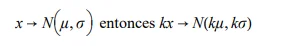21 Mar 2023

Posted on:

14 Feb 2022

1

# Resolved:transforming random variables

why does the distribution shrink when we multiply ? we are scaling up so the variance should be more higher thus more spread

Instructor
Posted on:

15 Feb 2022

0

Hey,

Following the link, you will open a desmos graph where I have defined two functions - f(x) and g(x). Let me quickly guide you through the website. By clicking on the empty circle to the left of each function, you will see it displayed on the coordinate system. You are able to display multiple graphs on the same plot. Using the scroller on your mouse, you can zoom in and out on the coordinate system.

Let me first discuss the function f(x) = x^2. The resulting graph is a parabola. Here are the coordinates of a couple of points on this parabola:
x = 1 -> f(x) = 1^2 = 1
x = 2 -> f(x) = 2^2 = 4
x = 3 -> f(x) = 3^2 = 9

Now, on the same coordinate system, let's display the function f(2x). It bears the following form:
f(2x) = (2x)^2 = 4x^2
What I did above was to replace the x from the previous example with a 2x. On the plot, you see that the resulting parabola is narrower than the first one. Here are the coordinates of a couple of points on this new parabola:
x = 1 -> f(2x) = 4*1^2 = 4*1 = 4
x = 2 -> f(2x) = 4*2^2 = 4*4 = 16
x = 3 -> f(2x) = 4*3^2 = 4*9 = 36

So, you see that for the same value of x, the y-value of the two parabolae is different. More specifically, for the same value of x, the y-value of the second parabola is higher than the y-value of the first one. This is why the second one turns out to be narrower than the first one.

Analogously, you can plot the function f(x/2) and argue for yourself why the resulting parabola is more spread out that the one of f(x).

As a second example, I have plotted the function g(x) which has the form of a normal distribution. Applying the same arguments and trying to calculate the y-values of the functions for the same values of x, try to explain why g(2x) is narrower than g(x) and why g(x/2) is more spread out :)

Kind regards,
365 Hristina

Posted on:

11 Nov 2022

1

Hi @Hristina!, just a comment to say a great link to desmos :)

Posted on:

16 Nov 2022

1``````I had the same doubt when consulting the video, and when searching other materials, I believe that both the explanation in the video and the one provided in this question are wrong.
Among the properties of the variance is that if Var(x)= sigma^2.
By multiplying the variable X by a constant K
Var(Kx)=K^2 sigma^2.
That is why if the absolute value of k is greater than 1 the dispersion increases (flatter graph), if the absolute value is less than 1 the dispersion decreases (more pointed graph) and if it is equal to 1 it remains the same.
I hope feedback to solve the doubt :)
``````
Posted on:

21 Mar 2023

0

I have the same question as Enrique